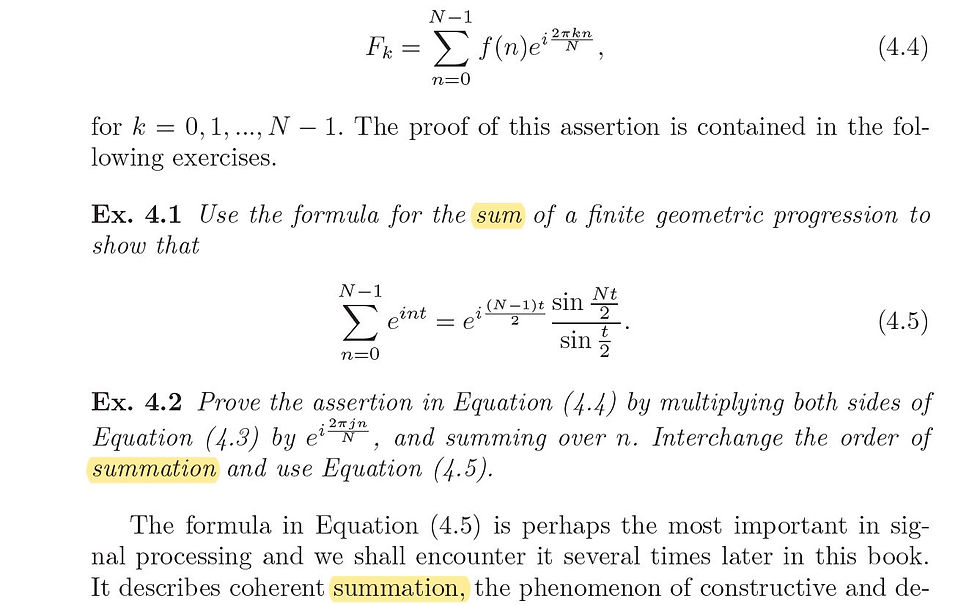top of page

# The Difference between "Sum" and "Summation" in Mathematical Writing"Sum" and "summation" in action. From "Introduction to the Theory of Numbers" by Hardy and Wright.

The difference between summation and sum should be clear enough: summation is the process of adding, whereas a sum is the result of the addition. Thus, "the sum of 1 + 2 + 3 + 4 + 5 is 15." The sum of the five numbers is 15 (the result). It'd be incorrect to write "the summation of the five numbers is 3."

This much is clear. Now, consider how the word summation is used. The summation operation is represented by the summation symbolThe addition 1 + 2 + 3 + 4 + 5 is represented in summation notation asHere, the variable i is the index of summation, and what is being summed is the index itself. Here is a more general summation:which representsAn important point is that the word sum can be used more flexibly than the simple distinction made in the first paragraph suggests. For example, instead of writing "Here is a more general summation" above, we could have written "Here is a more general sum" instead. It appears that sum can often be used in the same way as summation, which is what I think has misled some authors into thinking that the terms are synonyms; they then go on to make the error I highlighted in the first paragraph: using summation instead of sum to refer to the result of addition. If one of these authors were to consult a dictionary, he might see this (note definition 2):This author may well conclude that the terms are synonymous. No, sum and summation are not synonymous though sum can often replace summation.

Let's look at an example of how sum is used in Signal Processing: A Mathematical Approach by Charles Byrne:The first sum is a verb; the second and third instances are used for expressions defined by the summation operator.

Now let's look at another example from the same book:We see one sum and two summation's. Notice that the use of summation is general; the word is being used to mean "the process of summing." Notice also that summation in these two cases cannot be replaced by sum, which brings us to another observation: the noun sum usually needs an article, whereas summation does not need an article.

In the above example, can we replace sum with summation? We'd then get "Use the formula for the summation of a finite geometric progression to show that ..." No, this doesn't work; it's like saying "the summation of 1 + 2 is 3." We could, however, write "Use the following summation to show that ..." The preposition of next to summation in "summation of" indicates that what is meant is the result of the summation, i.e., the sum. As a tentative rule of thumb, I think we can say that sum is best used in specific contexts, and summation is best used in general contexts.

However, take a look at this:Why is summation used in one context and sum in a similar-looking context? I found this example in An Introduction to the Theory of Numbers by Hardy and Wright. Now, Hardy, the great British mathematician, was also an excellent writer (e.g. A Mathematician's Apology). One can safely assume that his choice of summation in one place and sum in another was deliberate. Throughout the book, Hardy consistently refers to expressions defined by the summation operator as summations, an example being the first summation in the above example. But what about "sum" in "the last sum tends to 0 ..."? Well, here he uses sum because he's referring to the result of the summation.

To summarize:

(1) Summation means the process of summing, and sum means the result of summing.

(2) Summation is general and conceptual, whereas sum is concrete (but see (4) below).

(3) Beware of the construction "the summation of ..."; it is likely to be wrong. The preposition "of" indicates that what is meant is the result of the summation, i.e., the sum. (Of course, "let us now return to the summation of the type discussed in Chapter 5" is fine.)

(4) An expression defined by the summation operator can be referred to as either a sum or a summation. We've seen that Byrne preferred sum, whereas Hardy preferred summation. Whatever you choose, be consistent.

(5) The result of a summation (or sum!) is always referred to as a sum.

To conclude, let's take a few more examples:

(a) "... the received signal, which is the summation of the desired and delayed signals ... ". Applying Rule (3) above, we see that "summation" is incorrect here.

(b) "The summation of the degrees of any graph is even." This is the same as (a); "summation" is incorrect.

(c) "The summation extends over all the lattice points inByrne would use sum here; both are acceptable, but the sentence is from Hardy's book. Hardy uses "summation" consistently in such contexts in his book.

(d) "Consider the function f defined by the infinite sumHardy would choose summation here; both are acceptable, but Byrne (from whose book this sentence has been adapted) prefers sum. He uses sum consistently in such contexts in his book.

Hardy restricts sum to the result of a summation and uses summation for pretty much everything else, including for expressions defined by the summation operator. Byrne, on the other hand, in addition to using sum for the result of a summation, also uses it for expressions defined by the summation operator. Whichever convention you adopt, apply it consistently to avoid confusing the reader.

Yes, you can label this discussion an exercise in hairsplitting, but both academic copyeditors and mathematicians spend a lot of their time splitting hairs. It's important to be precise in academic writing.